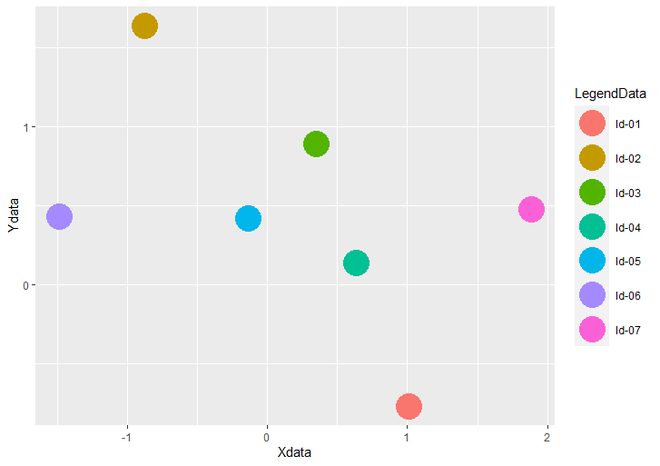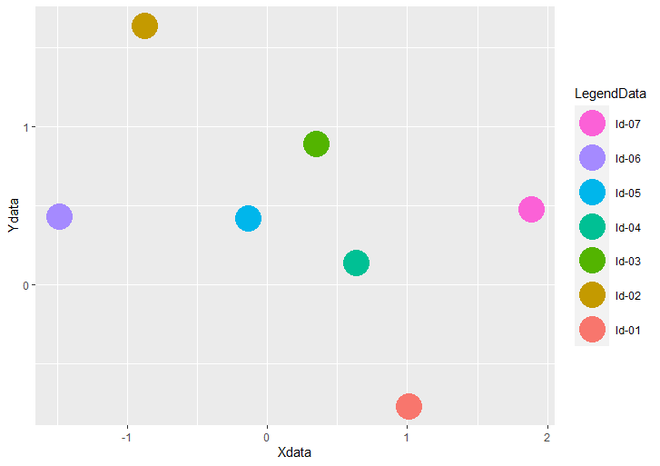Related Articles

# Reversing the order of a ggplot2 legend

• Last Updated : 02 Jul, 2021

In this article, we will see how to Reverse the order of Legend Items of ggplot2 plots in the R programming language.

### Getting Started

First, load the ggplot2 package by using the library() function. If you have not installed it yet, you can simply install it by writing the below command in R Console.

`install.packages("ggplot2")`

Let us first create a regular plot, so that the difference is apparent.

Example:

## R

 `# Load Package``library``(``"ggplot2"``)` `# Create a DataFrame``data <- ``data.frame``(Xdata = ``rnorm``(7),                       ``                   ``Ydata = ``rnorm``(7),``                   ``LegendData = ``c``(``"ld-01"``, ``"ld-02"``,``                                  ``"ld-03"``, ``"ld-04"``,``                                  ``"ld-05"``, ``"ld-06"``,``                                  ``"ld-07"``))` `# Create a Scatter Plot``ggplot``(data, ``aes``(Xdata, Ydata, color = LegendData)) +  ``  ``geom_point``(size = 10)`

Output:Scatterplot with legend in Default Order

To Reverse the order of Legend, we have to add guides() and guide_legend() functions to the geom_point() function. Inside guides() function, we take the parameter color, which will call guide_legend() guide function as value. Inside guide_legend() function, we take an argument called reverse, which is a logical parameter. If “reverse = TRUE”, the order of legends is reversed otherwise it will remain as it was.

Syntax : guides(…)

Parameter :

• … : either a string or call to a guide function. here we call guide_legend() guide function.

Return : each scale can be set scale-by-scale

Syntax : guide_legend(reverse = TRUE)

Parameter :

• reverse : It is a logical parameter that specify the order of plot legend.

Return : Legend Guides for various scales

Example:

## R

 `# Load Package``library``(``"ggplot2"``)` `# Create a DataFrame``data <- ``data.frame``(Xdata = ``rnorm``(7),                       ``                   ``Ydata = ``rnorm``(7),``                   ``LegendData = ``c``(``"ld-01"``, ``"ld-02"``,``                                  ``"ld-03"``, ``"ld-04"``,``                                  ``"ld-05"``,  ``"ld-06"``,``                                  ``"ld-07"``))` `# Create a Scatter Plot and change``# the size of legend``ggplot``(data, ``aes``(Xdata, Ydata, color = LegendData)) +  ``  ``geom_point``(size = 10)+``  ``guides``(color = ``guide_legend``(reverse=``TRUE``))`

Output:Scatterplot with legend in Reverse Order

My Personal Notes arrow_drop_up